# Electronics and Communication Engineering - Electromagnetic Field Theory

1.
ZL = 200 Ω and it is desired that Zi = 50 Ω The quarter wave transformer should have a characteristic impedance of
100 Ω
40 Ω
10000 Ω
4 Ω
Explanation:

Z0 = Zi . ZL

2.
A broadside array consisting of 200 cm wavelength with 10 half-wave dipole spacing 10 cm. And if each array element feeding with 1 amp. current and operating at same frequency then find the half power beamwidth
10°
15°
Explanation: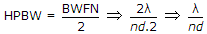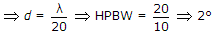.

3.
Refractive index of glass is 1.5. Find the wavelength of a beam of light with a frequency of 1014 Hz in glass. Assume velocity of light is 3 x 108 m/sec in vacuum.
4 μm
3 μm
2 μm
1 μm
Explanation:

Given, μ = 1.6, f = 1014 Hz, v = 3 x 108 m/sec.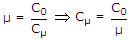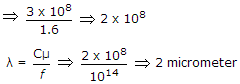4.
The function f(x - vot) represent which of the following?
A stationary wave
A wave motion in forward direction
A wave motion in reverse direction
None of the above
Explanation:

f(x - vot) represents in +ve direction while f(x + vot) represents a progressive wave in -ve direction.

5.
A broadside array operating at 100 cm wavelength consist of 4 half-wave dipoles spaced 50 cm apart. Each element carries radio frequency current in the same phase and of magnitude 0.5 A. The radiated power will be
196 W
73 W
36.5 W
18.25 W
P = n . I2 . Rr, where Rr = 80p2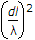Ω.